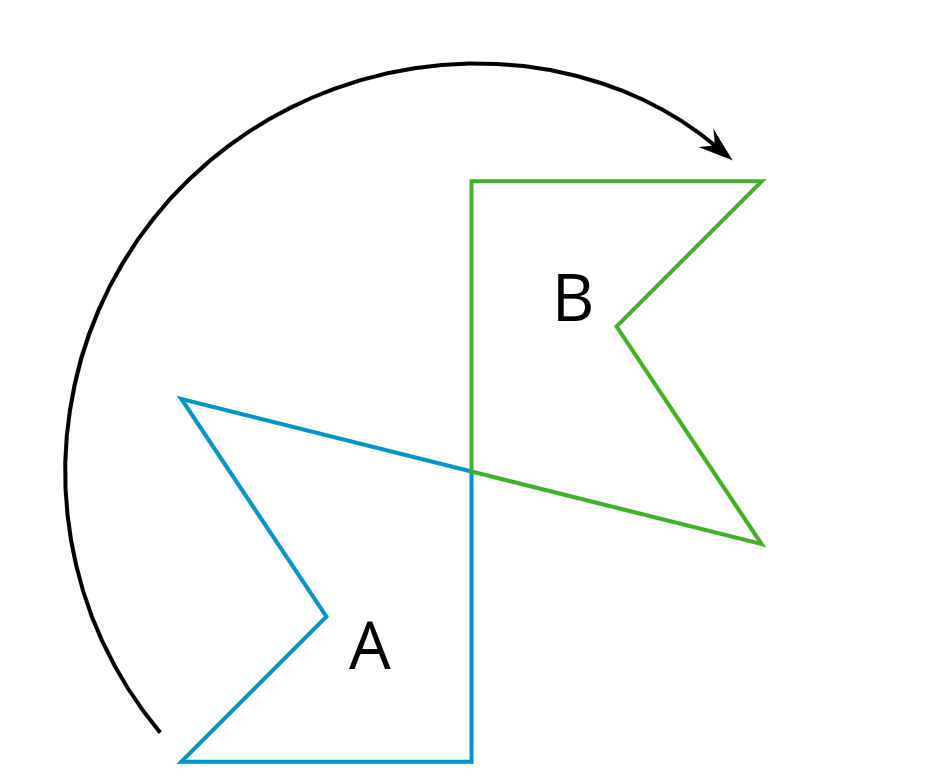# Lesson 3

Grid Moves

### Lesson Narrative

Prior to this lesson, students have learned the names for the basic moves (translation, rotation, and reflection) and have learned how to identify them in pictures. In this lesson, they apply translations, rotations, and reflections to figures. They also label the image of a point $$P$$ as $$P'$$. While not essential, this practice helps show the structural relationship (MP7) between a figure and its image.

Students also encounter the isometric grid (one made of equilateral triangles with 6 meeting at each vertex). They perform translations, rotations, and reflections both on a square grid and on an isometric grid. Expect a variety of approaches, mainly making use of tracing paper (MP5) but students may also begin to notice how the structure of the different grids helps draw images resulting from certain moves (MP7).

For classrooms using the digital version of the materials: This is the lesson where students learn to use the transformation tools in Geogebra.

### Learning Goals

Teacher Facing

• Describe (orally) the moves needed to perform a transformation.
• Draw and label the image and “corresponding points” of figures that result from translations, rotations, and reflections.
• Draw the “image” of a figure that results from a translation, rotation, and reflection in square and isometric grids and justify (orally) that the image is a transformation of the original figure.

### Student Facing

Let’s transform some figures on grids.

### Required Preparation

Make sure students have access to items in their geometry toolkits: tracing paper, graph paper, colored pencils, scissors, ruler, protractor, and an index card to use as a straightedge or to mark right angles.

For classrooms using the print version of the materials: Access to tracing paper is particularly important. Each student will need about 10 small sheets of tracing paper (commercially available "patty paper" is ideal).  If using large sheets of tracing paper, such as 8.5 inches by 11 inches, cut each sheet into fourths.

For classrooms using the digital version of the materials: If you have access to extra help from a tech-savvy person, this would be a good day to request their presence in your class.

### Student Facing

• I can decide which type of transformations will work to move one figure to another.
• I can use grids to carry out transformations of figures.

Building On

Building Towards

### Glossary Entries

• clockwise

Clockwise means to turn in the same direction as the hands of a clock. The top turns to the right. This diagram shows Figure A turned clockwise to make Figure B.• counterclockwise

Counterclockwise means to turn opposite of the way the hands of a clock turn. The top turns to the left.

This diagram shows Figure A turned counterclockwise to make Figure B.

• image

An image is the result of translations, rotations, and reflections on an object. Every part of the original object moves in the same way to match up with a part of the image.

In this diagram, triangle $$ABC$$ has been translated up and to the right to make triangle $$DEF$$. Triangle $$DEF$$ is the image of the original triangle $$ABC$$.

• reflection

A reflection across a line moves every point on a figure to a point directly on the opposite side of the line. The new point is the same distance from the line as it was in the original figure.

This diagram shows a reflection of A over line $$\ell$$ that makes the mirror image B.

• rotation

A rotation moves every point on a figure around a center by a given angle in a specific direction.

This diagram shows Triangle A rotated around center $$O$$ by 55 degrees clockwise to get Triangle B.

• translation

A translation moves every point in a figure a given distance in a given direction.

This diagram shows a translation of Figure A to Figure B using the direction and distance given by the arrow.

The vertices in this polygon are labeled $$A$$, $$B$$, $$C$$, $$D$$, and $$E$$.